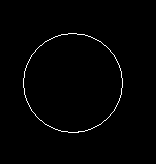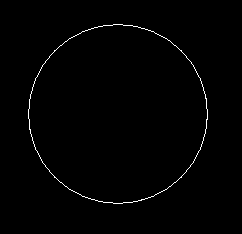GFG App
Open AppBrowser
Continue

# Draw circle in C graphics

The header file graphics.h contains circle() function which draws a circle with center at (x, y) and given radius.

Syntax :

```circle(x, y, radius);

where,
(x, y) is center of the circle.
```

Examples :

```Input : x = 250, y = 200, radius = 50
Output :Input : x = 300, y = 150, radius = 90
Output :```

Below is the implementation to draw circle in C:

 `// C Implementation for drawing circle ` `#include ` ` `  `//driver code ` `int` `main() ` `{ ` `    ``// gm is Graphics mode which is ` `    ``// a computer display mode that ` `    ``// generates image using pixels. ` `    ``// DETECT is a macro defined in ` `    ``// "graphics.h" header file ` `    ``int` `gd = DETECT, gm; ` ` `  `    ``// initgraph initializes the ` `    ``// graphics system by loading a ` `    ``// graphics driver from disk ` `    ``initgraph(&gd, &gm, ``""``); ` ` `  `    ``// circle function ` `    ``circle(250, 200, 50); ` ` `  `    ``getch(); ` ` `  `    ``// closegraph function closes the ` `    ``// graphics mode and deallocates ` `    ``// all memory allocated by ` `    ``// graphics system . ` `    ``closegraph(); ` ` `  `    ``return` `0; ` `} `

Output :

``````
My Personal Notes arrow_drop_up